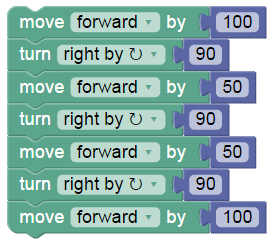# Computer Science Programming Progress Banding

Develop Programs

### Develop Programs

• The student demonstrates the ability to develop simple, sequential programs that solve a variety of programming problems.
• The solution may not be an efficient solution to the problem
• The solution may not fully work as intended
• The solution may not use sensible variable names

Example 1 – Draw a triangle.The solution solves the problem, but not very efficiently.

Example 2 – Ask for user input, adding two numbers and display the output.The solution solves the problem, but does not use sensible variable names.

Debug & Test Programs

Debug & Test Programs

Students can spot simple mistakes in sequential programs.

Examples

Example 1The following code should make a rectangle, what error has been made?

Evaluate Programs

Discuss Programs

Examples